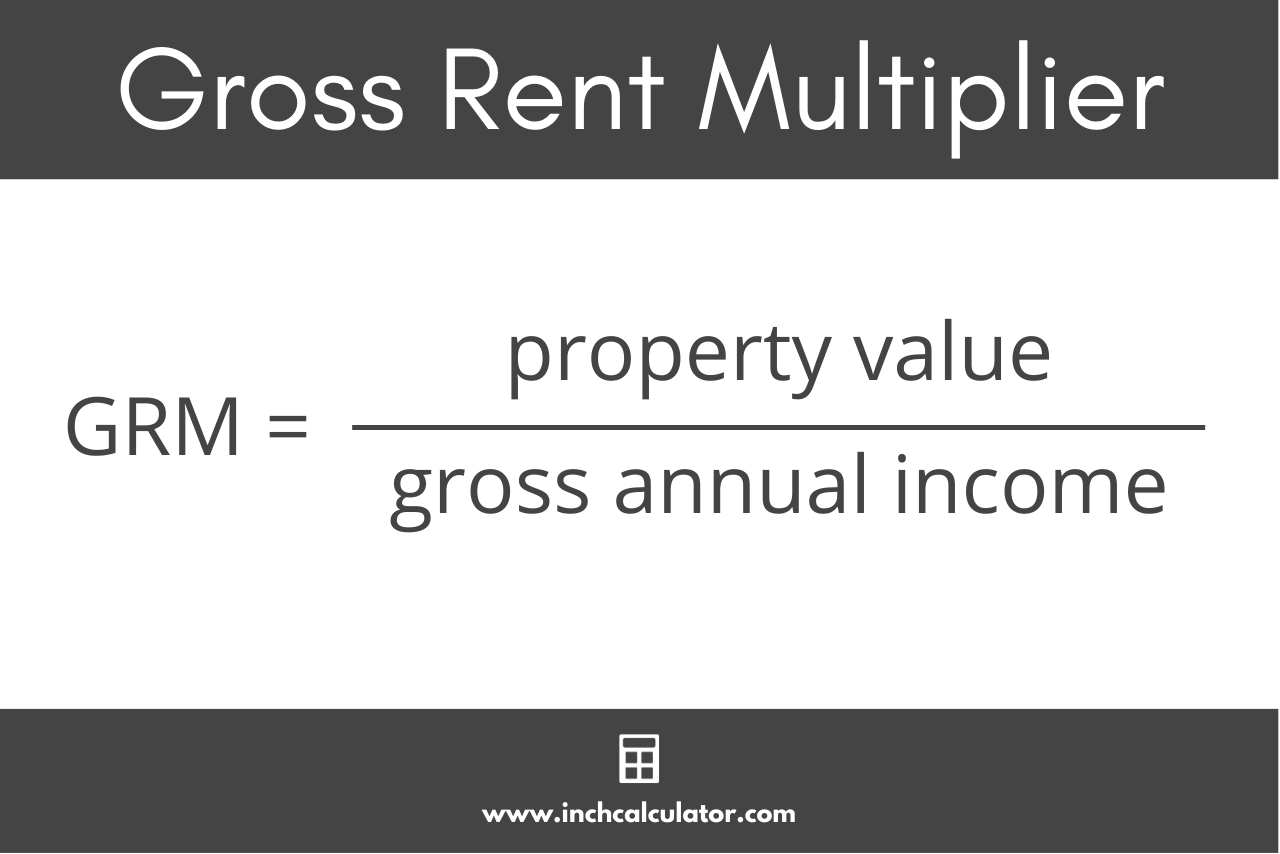# Gross Rent Multiplier Calculator

Use the calculator below to find the gross rent multiplier for a property. Learn how to use the GRM formula below.

\$
\$

## Gross Rent Multiplier:

Learn how we calculated this below

## How to Find the Gross Rent Multiplier

The gross rent multiplier (GRM) is a metric commonly used by real estate investors to determine a property’s potential return on investment. The gross rent multiplier is a ratio of the property value or purchase price to its annual gross rental income.

You can calculate it using the gross rent multiplier formula below.

### Gross Rent Multiplier Formula

gross rent multiplier = property value / gross annual income

To calculate the GRM, divide the property’s value by the gross income it generates. To use this formula, you will need the property value (or purchase price) and its gross annual rental income.For example, let’s find the gross rent multiplier for a property valued at \$500,000 that generates \$25,000 in gross rental income annually.

gross rent multiplier = \$500,000 / \$25,000 = 20.00

So, the GRM for this property is 20.00, meaning it will take 20 years of rental income to recoup the property’s value.

## Interpreting the Gross Rent Multiplier

The gross rent multiplier is a quick way to compare the value of different properties. A lower GRM indicates that the property generates more income relative to its purchase price, making the payoff period for the loan or investment quicker.

While it is an easy calculation, the gross rent multiplier doesn’t account for any expenses related to the property, such as taxes, insurance, or maintenance costs.

It’s just one metric used to compare properties relative to each other, but other metrics, such as the cap rate, may be more useful since it uses the net operating income rather than the gross income.